## What is The Difference Between Wiring Batteries in Series Vs. Parallel?

Series vs. parallel wiring: do you know the difference? If not, do not worry – this blog post will explain everything. We will also demonstrate how to wire your batteries in each configuration and discuss the advantages of each style of wiring so you can determine which is best for you. Let us get started!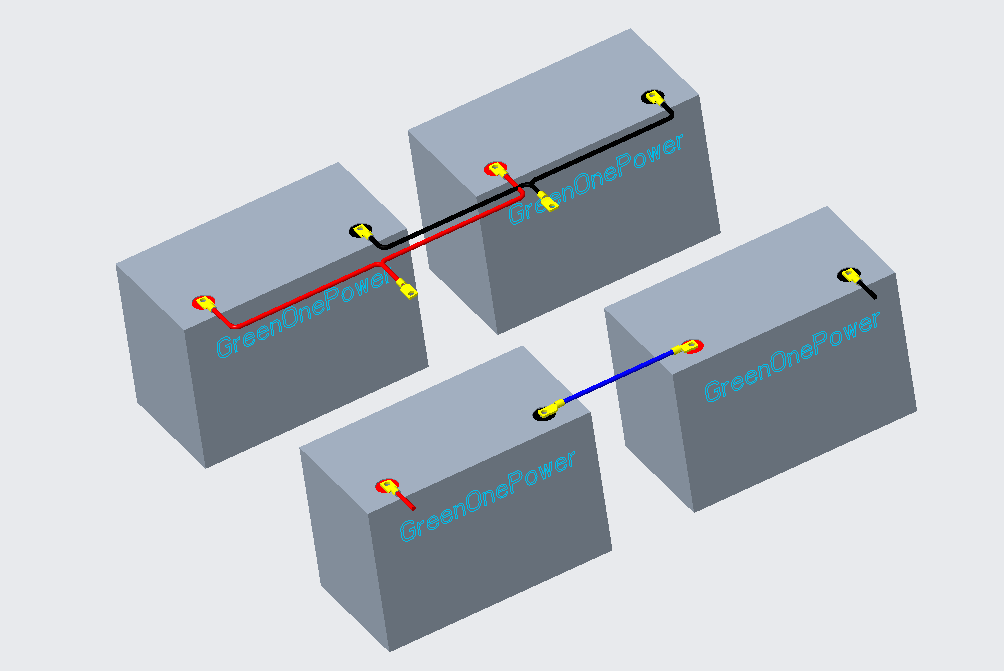### What is the distinction between wiring batteries in series and parallel?

The two most common ways to wire batteries are in series and parallel. Both configurations have their advantages and disadvantages, depending on the desired outcome.

When wiring Lithium batteries in series, the voltages of the individual batteries are added together, this is useful for applications where a higher output voltage is required, such as in an RV or boat where 12 volts is standard. However, the system’s capacity is reduced because only one battery supplies power at a time.

In contrast, wiring batteries in parallel keeps the voltage the same but increases the capacity. This is helpful when a long run time is necessary, such as in a wheelchair or other powered device.

However, it should be noted that the total available energy in both configurations is equal. That means that while one may be able to run for a longer period with Lithium batteries wired in parallel, the amount of power that can be delivered at any given moment is still limited by the number of batteries in the system.

For example, let us say you have two 12-volt, 100 amp hour batteries. You can make a 24-volt, 100-amp-hour battery by connecting them in series. However, if you wire them in parallel, you will still have a 12-volt, 200-amp hour battery.

### Wiring Batteries in Series

To wire multiple batteries in series, connect each battery’s positive terminal to the next negative terminal. Then, in series, measure the total output voltage of the system between the negative terminals of the first and last batteries. Let us look at two examples to make this clear.

##### Wiring Batteries In 12V Series Configuration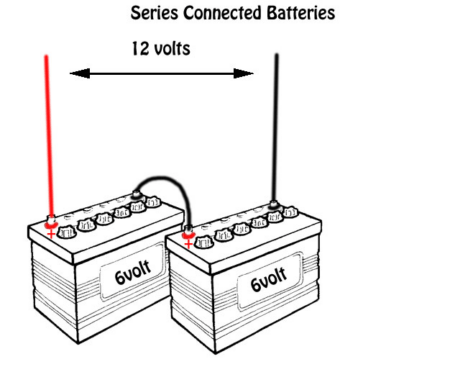The wiring configuration mentioned below needs two 6V batteries. You will be able to derive 12V from the farthest positive and negative post by utilizing any two 6V batteries that are wired in a positive-negative setup. Your load should be connected at this point.

##### Wiring Batteries In 48V Series Configuration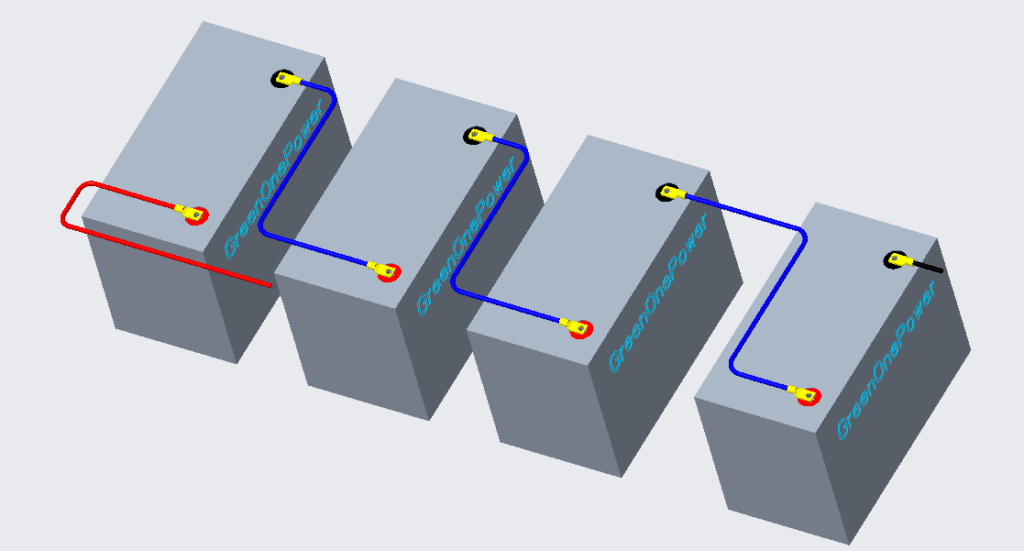You will need four 12V batteries for the following wiring configuration. Connecting any four 12V Lithium batteries, positive to negative, will create 48 volts on the farthest negative and positive post. This is where you will connect your load.

###### Current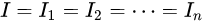For a series circuit, the current is uniform for all components.

###### Voltage

The voltage in a series circuit is the sum of the component voltages, which cause voltage drops (resistance units).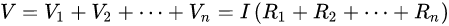###### Resistance units

Two or more resistors connected in series have a total resistance equal to the sum of their resistances.The subscript s in Rs indicates “series,” while Rs refer to a series’s resistance. The electrical conductance is equal to the reciprocal of resistance. The following equation may be used to calculate the total conductance of a series of circuits of pure resistances: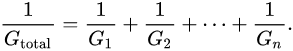In this case, the total conductance is equal to. For a special case of two conductances in a series,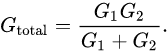• One advantage of wiring batteries in series is that it increases the total voltage. This is handy if you need to power a gadget that demands more voltage than a single battery can supply. For example, if you have four 12-volt batteries, you may connect them in series to generate a 48-volt power supply.
• One of the main disadvantages of wiring batteries in series is that it limits the voltages that can be used on the system. Lower voltages cannot be obtained from a battery bank in a series-connected system without using a converter. To utilize 12V appliances on the system, all equipment must operate at a higher voltage or an extra converter. This may be expensive and cumbersome, particularly if many devices demand different voltages.
• Additionally, wiring batteries in series can decrease the system’s overall capacity. This is because each battery in the series effectively shares its capacity with the other batteries in the circuit. As a result, wiring batteries in series is not always the best option for maximizing power and efficiency.

### Wiring Batteries in Parallel

You can wire multiple batteries in parallel by connecting all of the positive terminals and all of the negative terminals. This method can measure the system output voltage across any positive and negative battery terminals since they are all connected. Let us look at several instances to help you understand.

### Wiring Batteries In 100Ah Parallel Configuration

For instance, two 100 Ah batteries at 6 volts wired in parallel have a total system voltage of 6 volts and a capacity of 200 Ah. The positive terminal on one battery is connected to the positive terminal on the other, and both negative terminals are also connected.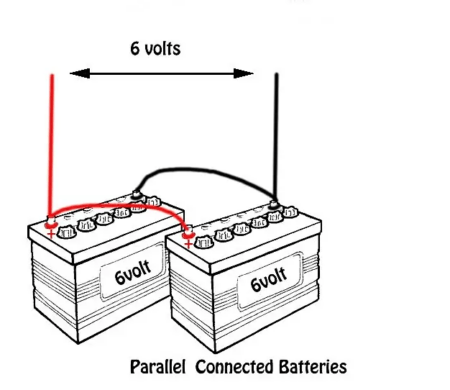##### Wiring Batteries In 400Ah Parallel Configuration###### Voltage

In a parallel circuit, the voltage is the same across all wires.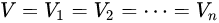###### Current

Ohm’s law can find the current in every resistor. The voltage cancelling out gives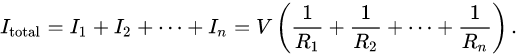###### Resistance units

It is easy to determine the total resistance of all components by adding up the reciprocals of each component’s resistances Ri and taking the sum’s reciprocal. The value of the smallest resistance will always be less than that of any other resistance: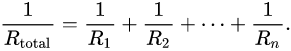Two different resistances are required for the unreciprocated statement to be simple: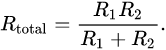The reciprocal sum expression for N parallel resistances simplifies to: If you want to understand more about how this works, have a look at my series on interpreting reciprocals.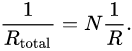And therefore, to: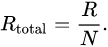To find the current, or amount of electricity moving through a component per unit time, with resistance Ri in a circuit, use Ohm’s law: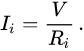The components in the circuit divide the current evenly according to their reciprocal resistances. In other words, if there are only two resistors present in the circuit,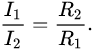A parallel circuit of resistors is also known as multiple, such as a group of arcs connected in parallel. Because electrical conductance G is proportional to resistance, the following formula for total conductance of a parallel circuit of resistors holds: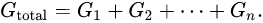The total conductance and resistance have a complementary relationship – the equation for series resistances is the same as for parallel conductances, and vice versa.

• One of the primary benefits of putting batteries in parallel is that you may expand your system’s possible runtime while preserving voltage. This is because the amp-hour capacities are additive, so two batteries in parallel double your runtime, three triple it, and so on. This can be beneficial if you need to operate a system for an extended period of time or know you will be without access to a power source for an extended period of time.
• Another benefit of connecting batteries in parallel is that if one of your batteries dies or has a problem, the remaining batteries in the system can still give power. This is helpful because it means you will not be left without power if something happens to one of your batteries. You will be able to utilize the others until you can replace or repair the broken one.

Overall, wiring batteries in parallel has many advantages that can be useful in different situations. If you need a long runtime or want to ensure you will not be left without power, wiring batteries in parallel is the way to go.

There are a few disadvantages to wiring batteries in parallel vs. series.

• One is that the system voltage will be lower, resulting in a higher current draw. This can make operating larger power appliances and generation difficult, as they are less efficient when operating at lower voltages.
• Another drawback is that thicker cables may be needed to handle the higher current, leading to more voltage drops.
• Finally, wiring batteries in parallel can create an imbalance if one battery drains faster than the others, as this can cause the system to shut down.

### Can You Wire Batteries in Series and Parallel?

When you wire batteries in series, you connect them end to end, positive to negative, so that the battery’s voltage is added together. This is why you cannot wire the same batteries in series and parallel, as doing so would create a short circuit. However, you can wire sets of batteries in series and parallel to create a larger battery bank at a higher voltage.

For example, if you have two sets of 12-volt batteries, you can wire them in series so that they create a 24-volt battery bank. You can then wire these two sets in parallel so that they create a battery bank with a higher capacity. The graphic below demonstrates how this may be performed.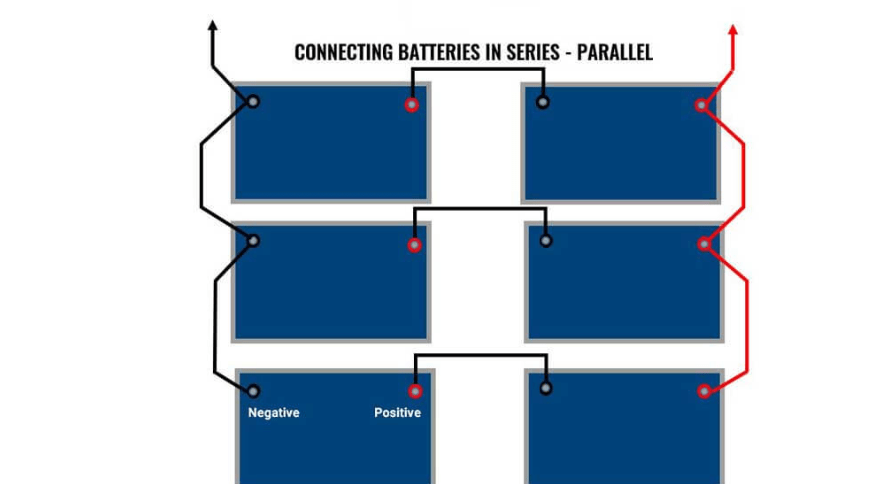As you can see, each set of batteries is wired in series and parallels to create a battery bank. These two banks are then wired in parallel to create a single battery bank with a high voltage and capacity. By wiring batteries this way, you can create a large and powerful battery bank that can be used for various applications.

### Are you curious about electrical systems and lithium batteries?

If you are concerned about an electrical project, please do not hesitate to contact our staff. We at Green ONE Power would be delighted to give support and answer any queries you may have. You can reach out to us at (86) 191 2956 3884.